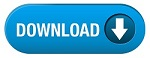Home » SSC » Model Questions for Scientific Assistant (IMD) Exam (Paper-2) PHYSICS – Set 27

# Model Questions for Scientific Assistant (IMD) Exam (Paper-2) PHYSICS – Set 27

a. 680 m/s
b. 6800 m/s
c. 340 m/s
d. 3400 m/s

## 2. You hear a lot of static from your radio. Is the static you hear more likely to result from receiving FM or AM radio waves?

a. FM
b. AM
c. Neither produces any static.
d. Both produce equal amounts of static.

a. 34 meters
b. 3.4 meters
c. 340 meters
d. 3.4 km

## 4. Measured in volts. This is the difference in electric potential between two points.

a. Potential Difference
b. Electric Current
c. Electrical resistance
d. Superconductor

## 5. Measured in ohms. A material resists the flow of electric charge, while permitting some flow of electric charge.

a. Potential Difference
b. Electric Current
c. Electrical resistance
d. Superconductor

### 6. Current in a circuit increases if voltage is increased, and decreases if resistance is increased.

a. Potential Difference
b. Electric Current
c. Electrical resistance
d. Ohm’s law

## 7. Electrical charges flow in one direction, then switch direction and flow in the opposite direction. They repeat this process, usually several times a second.

a. Alternating current (AC)
b. Electric power
c. Series ciruit
d. Parallel circuit

## 8. Measured in amperes. Electric charges flow and transport energy from one location to another.

a. Potential Difference
b. Electric Current
c. Electrical resistance
d. Superconductor

## 9. At very low temperatures, these materials exhibit no resistance against current flow.

a. Superconductor
b. Ohm’s law
c. Direct current (DC)
d. Alternating current (AC)

## 10. Electric current flowing in only one direction.

a. Direct current (DC)
b. Alternating current (AC)
c. Electric power
d. Series ciruit

#